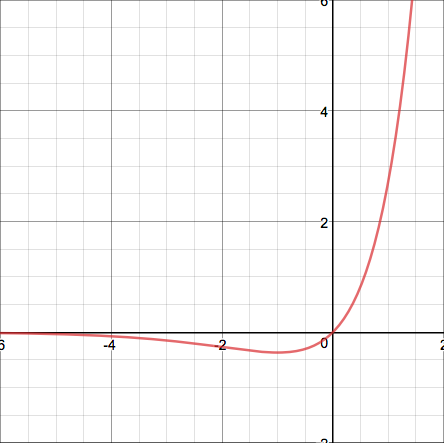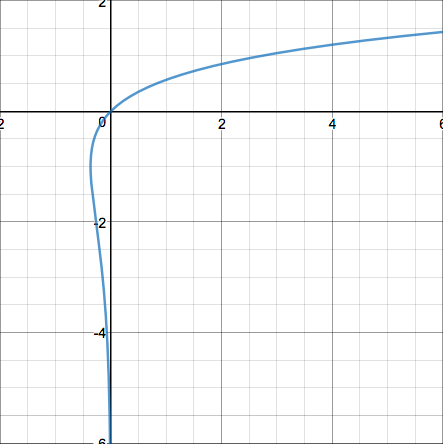## The Gamma and Lambert-W Functions

2017 June 21

Find the values of the following integrals, where $W(x)$ denotes the inverse function of $y=xe^x$ from $0$ to $\infty$:

I will be devoting this post to two very interesting and related functions: the Gamma Function $\Gamma(z)$ and the Lambert-W Function $W(z)$.

I will start off with the basics of the Lambert-W function. This function is taken to be the inverse function of $y=xe^x$. Here are two graphs, one of $y=xe^x$ (in red), and one of the Lambert-W function (in blue).As you can see, $y=xe^x$ is not injective and should not have an inverse, but because only a small branch of it is non-injective, it can be useful to treat is as if it had an inverse anyways. This is what the Lambert-W function is for. That function cannot be inverted anyways using elementary functions, so the Lambert-W function is what we treat as its inverse. However, since it is not actually injective, the Lambert-W function has two branches: the lower branch $W_{-1}$ and the upper branch $W_0$. The point at which the function changes between these two branches is at the point corresponding to the minimum of $y=xe^x$, the point $(-\frac{1}{e},-1)$ on the Lambert-W function. Because it is defined as the inverse of $y=xe^x$, it has the properties

The value $W(1)$, or the unique solution to the equation is called the omega constant $\Omega$ and is about $0.5671$. We will be using it in some of our later integral problems.

This function can be used to "solve" many new types of equations. For example, consider the equation This equation can be solved using the Lambert-W function in the following way: There are two possible values satisfying this, since both branches of the Lambert-W function exist at $x=-\frac{1}{3}$.

Here's another example: This can be solved in the following way:

The derivative of the Lambert-W function is given by I will not go into the details of the derivation of this formula, because it can be attained easily using the formula for the derivative of the inverse of a function, in this case the function $f(x)=xe^x$. Furthermore, its antiderivative is which can also be obtained with the use of a formula.

We will now derive two identities of the Lambert-W function that we will now prove that may become helpful later. The first is the sum identity and the second is the product identity Here is the derivation of the first identity: And here is the derivation of the second:

Before we move into the "good stuff", it would be best to introduce the Gamma Function, as it can save a lot of time when evaluating some of the integrals that we will later evaluate.

Basically, the Gamma Function is an extension of the factorial function to non-natural numbers. It has the property So it is essentially the factorial function, translated one unit. It is defined as Its relation to the factorial function can be proven using induction. First we must show that $\Gamma(1)=1$:

Wonderful. Now we must begin the inductive part of the proof. If we use integration by parts, we can observe that Which means that Which completes our inductive proof.

One thing that is helpful when working with the Gamma Function is knowledge of the Gaussian Integral; that is, the integral This integral comes up often when evaluating particular values of the Gamma Function. For example, look what happens when we try to evaluate $\Gamma\bigg(\frac{1}{2}\bigg)$: If we make the substitution $x \to y^2$, this integral turns into Which is just the same as the Gaussian Integral, and so

I'll spare you the derivations of the first couple values of this type of the Gamma Function, as they each involve a lot of integration by parts and are very repetitive. Here they are:

Are you noticing a pattern?

Do you see it? This can lead us to conjecture that

This can be proven easily using induction. This proof is also inductive and also uses integration by parts. The first step is recalling that $\Gamma(\frac{1}{2})$ is equal to $\sqrt{\pi}$. Then we can begin our inductive step. By definition, And if we use integration by parts, we notice that Meaning that Which completes the induction step of our proof.

It can be proven similarly that where $!_k$ represents the kth factorial (that is, $!_2$ is $!!$, $!_3$ is $!!!$, and so on). This fact will be useful to us later on.

One final notable formula regarding the Gamma Function is its reflection formula, derived by Euler:

However, we will not use it much, and so we will not derive it here. Perhaps in a later post that is focused solely on the Gamma Function.

Before we start the integrals, let me remind you of the definite integral property Because we will be using it a lot in the upcoming problems.

First off, we will tackle the least intimidating of the integrals: It seems impossible at first to integrate over a function that cannot even be expressed using other elementary functions. However, we can use the trick that I just mentioned, along with the fact that $W(x)$ is defined as the inverse of $xe^x$. Let's use the trick with $g(x)=xe^x$. Then we get $W(0)$ is $0$ and $W(1)$ is the omega constant, so we can now simplify the integral and the rest of the bounds: Using integration by parts, we can reduce this to Remember, since $\Omega=W(1)$, we can simplify $\Omega e^\Omega$ to $1$: Furthermore, since $\Omega e^\Omega=1$, then $e^\Omega=\frac{1}{\Omega}$, so our simplified answer is and so

On to the next one: Let us again use our "trick" with $g(x)=xe^x$: Now the integral can be solved readily by using integration by parts over and over again. I'll spare you the details:

Now for the integral This time we can use $g(x)=-\ln(xe^x)=-\ln(x)-x$ to get the much easier integral and so

Next up is Let us once again use $g(x)=xe^x$ to get Now we can recognize the relevance of the gamma function. If we use $g(x)=2x$ then we get and, since we have already obtained these values for the Gamma Function, we have and so

Now for the final integral: First we will use $g(x)=\sqrt{\frac{1}{x}e^{-x}}$, which gives us Now let us use $g(x)=2x$: and so

And that concludes this blog post!